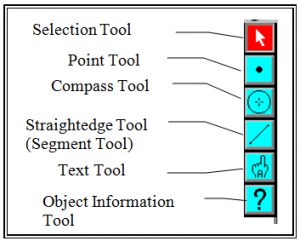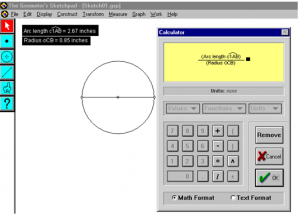# How Far is it Around that Circle?

//How Far is it Around that Circle?

## How Far is it Around that Circle?

Materials:  Compass, ruler, paper, string, Geometer’s Sketchpad.

### Activity 1:

1. Open your compass approximately 2 inches and construct a circle.
2. Without changing the opening of your compass, mark off equal lengths around the outside of the circle.
3. Draw segments between consecutive marks on the circle.
4. What type of figure have you constructed? _____________________
5. Do the last mark and the first mark you made perfectly coincide? __________________
6. Using a string, make a circle that’s congruent to the circle on your paper.
7. Measure the length of string needed to make the circle above.  ___________________
8. Measure the length of the diameter of your original circle. _____________________
9. What is the ratio of the length of the string and the diameter of the circle? __________________

### Activity 2:

To get a more precise measurement, we will use a computer program called The Geometer’s Sketchpad.

1. Start The Geometer’s Sketchpad.2. Choose the segment tool.
3. Draw a segment by clicking on your page and dragging. This will be the diameter of the circle.
4. With the segment selected, click on the “Construct” menu, and choose a point at midpoint.  In this step we have found the ___________ of the circle.
5. With the selection tool active, choose the midpoint and one end of the segment while holding down the shift key.  (The midpoint must be chosen first.)
6. From the Construct menu, choose circle by center and point.
7. Using the Selection tool, select the circle and both points on the diameter (hold down the shift key to select more than one object at a time.)
8. From the Construct menu, choose construct an arc on the circle.
9. With that arc chosen, click on the Measure menu, and then arc length.
10. Select the circle, and then click on the Measure menu, then radius.

### Further Investigation

1. Select the two measurements, then click on the Measure menu and choose calculate.2. From the Values button, choose Arc Length, then click on the “/” symbol.
3. From the Values button, choose Radius, then click on ok.
4. What is the ratio of the Arc Length to the Radius? ___________________
5. Grab a point on the circle and stretch the circle.
6. What is the ratio of the Arc Length to the Radius of any circle?
7. Select the circle and measure its area.
8. How is its area related to its radius?
9. Write a formula explaining this relationship. ________________
By | 2017-11-13T22:02:03+00:00 January 20th, 2015|Geometry|0 Comments

### About the Author: Stacie Bender